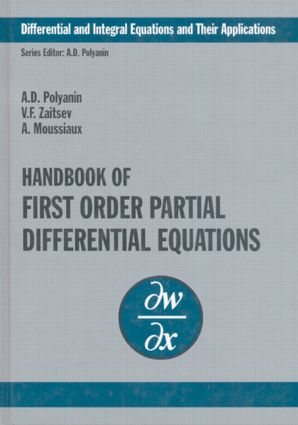Handbook of First-Order Partial Differential Equations

1st Edition

CRC Press

520 pages

Purchasing Options:\$ = USD
Hardback: 9780415272674
pub: 2001-11-15
SAVE ~\$55.00
\$275.00
\$220.00
x
eBook (VitalSource) : 9780429172960
pub: 2001-11-15
from \$28.98

FREE Standard Shipping!

Description

This book contains about 3000 first-order partial differential equations with solutions. New exact solutions to linear and nonlinear equations are included. The text pays special attention to equations of the general form, showing their dependence upon arbitrary functions. At the beginning of each section, basic solution methods for the corresponding types of differential equations are outlined and specific examples are considered. It presents equations and their applications, including differential geometry, nonlinear mechanics, gas dynamics, heat and mass transfer, wave theory and much more. This handbook is an essential reference source for researchers, engineers and students of applied mathematics, mechanics, control theory and the engineering sciences.

Linear Equations with Two Independent Variables. Linear Equations with Three or More Independent Variables. Nonlinear Equations. Equations with Two Independent Variables. Quadratic in Derivatives. Nonlinear Equations with Two Independent Variables or General Form Nonlinear Equations with Three or More Independent Variables. Supplement Solution of Differential Equations Through the CONVODE Software.